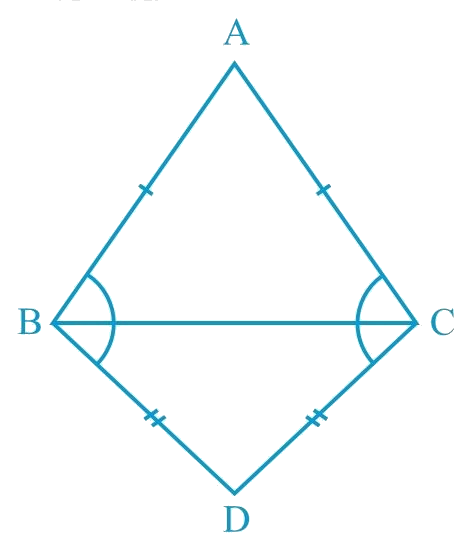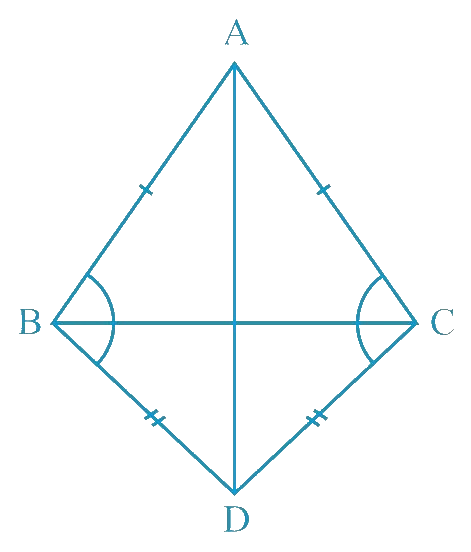# Ex.7.2 Q5 Triangles Solution - NCERT Maths Class 9

Go back to  'Ex.7.2'

## Question

$$ABC$$ and $$DBC$$ are two isosceles triangles on the same base $$BC$$ (see the given figure). Show that $$∠ABD = ∠ACD.$$

Video Solution
Triangles
Ex 7.2 | Question 5

## Text Solution

What is Known?

$$ABC$$ and $$DBC$$ are two isosceles triangles.

To prove:

$$\angle \text{ABD}=\angle \text{ACD}$$

Reasoning:

First of all, we can join point $$A$$ and $$D$$ then we can show two triangles $$ADB$$ and $$ADC$$ congruent by using SSS congruency rule after that we can say corresponding parts of congruent triangles will be equal.Steps:Let us join $$AD.$$

In $$\triangle ABD \text { and } \Delta ACD,$$

\begin{align}&AB = AC\;( \text {Given} ) \\ &BD = CD( \text {Given} )\\ &AD = AD \; (\text{Common side}) \\& \therefore \Delta ABD \cong \Delta ACD \\&(\text{By SSS congruence rule}) \end{align}

\begin{align}&\therefore \Delta ABD = \Delta ACD \;(\text{By }CPCT) \end{align}

Video Solution
Triangles
Ex 7.2 | Question 5

Learn from the best math teachers and top your exams

• Live one on one classroom and doubt clearing
• Practice worksheets in and after class for conceptual clarity
• Personalized curriculum to keep up with school